# According to Arrhenius equation rate constant K is equal towhich of the following options represents the graph of ln(K) vs 1/T? Option 1)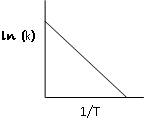Option 2)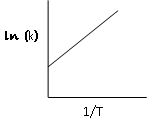Option 3)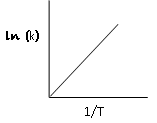Option 4)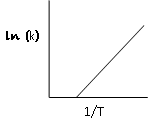As we learned in concept

Effect of Temperature and Activation Energy on rate constant -decreases with the increase in Tincreases with increase in T= Activation Energy

T = Temperature= Rate constant

- wherein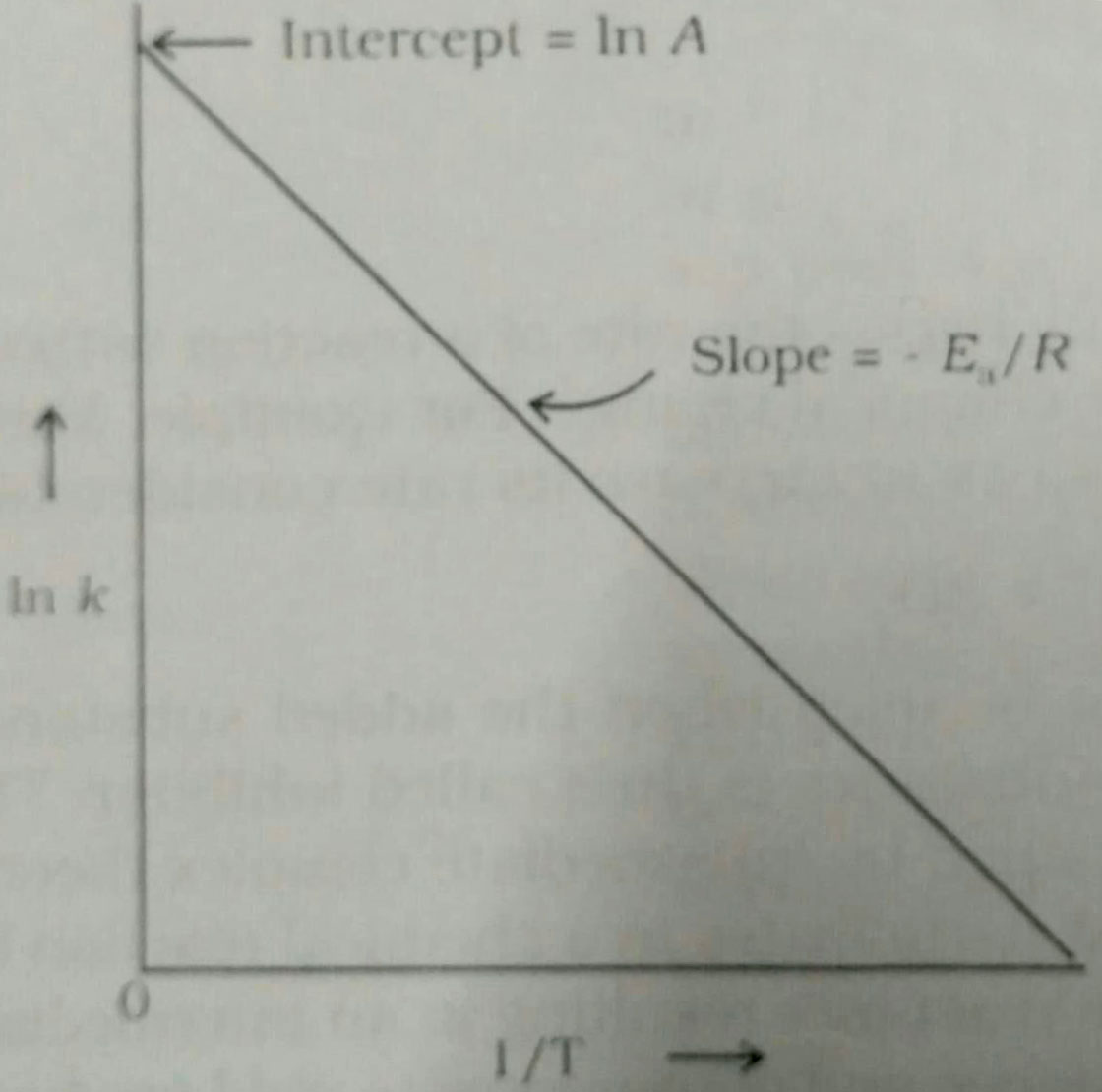Effect of Temperature and Activation Energy on rate constant -decreases with the increase in Tincreases with increase in T= Activation Energy

T = Temperature= Rate constant

- whereinArrhenius equation is given byThe graph of the above equaion can be drawn as follows:

Option 1)Correct option

Option 2)Incorrect option

Option 3)Incorrect option

Option 4)Incorrect option

Boost your Preparation for JEE Main 2021 with Personlized Coaching

Exams
Articles
Questions